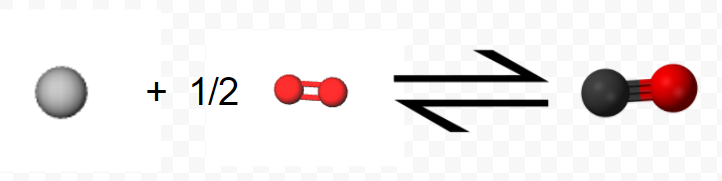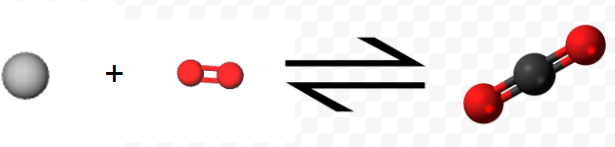# 3.9: Hess' Law

$$\newcommand{\vecs}{\overset { \rightharpoonup} {\mathbf{#1}} }$$ $$\newcommand{\vecd}{\overset{-\!-\!\rightharpoonup}{\vphantom{a}\smash {#1}}}$$$$\newcommand{\id}{\mathrm{id}}$$ $$\newcommand{\Span}{\mathrm{span}}$$ $$\newcommand{\kernel}{\mathrm{null}\,}$$ $$\newcommand{\range}{\mathrm{range}\,}$$ $$\newcommand{\RealPart}{\mathrm{Re}}$$ $$\newcommand{\ImaginaryPart}{\mathrm{Im}}$$ $$\newcommand{\Argument}{\mathrm{Arg}}$$ $$\newcommand{\norm}{\| #1 \|}$$ $$\newcommand{\inner}{\langle #1, #2 \rangle}$$ $$\newcommand{\Span}{\mathrm{span}}$$ $$\newcommand{\id}{\mathrm{id}}$$ $$\newcommand{\Span}{\mathrm{span}}$$ $$\newcommand{\kernel}{\mathrm{null}\,}$$ $$\newcommand{\range}{\mathrm{range}\,}$$ $$\newcommand{\RealPart}{\mathrm{Re}}$$ $$\newcommand{\ImaginaryPart}{\mathrm{Im}}$$ $$\newcommand{\Argument}{\mathrm{Arg}}$$ $$\newcommand{\norm}{\| #1 \|}$$ $$\newcommand{\inner}{\langle #1, #2 \rangle}$$ $$\newcommand{\Span}{\mathrm{span}}$$$$\newcommand{\AA}{\unicode[.8,0]{x212B}}$$

Perhaps the most useful feature of thermochemical equations is that they can be combined to determine ΔHm values for other chemical reactions. Consider, for example, the following two-step sequence. Step 1 is reaction of 1 mol C(s) and 0.5 mol O2(g) to form 1 mol CO(g):

$\text{C} (s) + \frac{1}{2} \text{O}_{2} (g) \rightarrow \text{C} \text{O} (g) ~~~~~~~~~~~~~~~~~~~~ \Delta H_{m} = –110.5 \text{kJ} = \Delta H_{1} \nonumber$Ball and stick structure shows 1 grey sphere reacting with half of double bonded 2 red spheres to give a triple bonded black and red sphere.

(Note that since the equation refers to moles, not molecules, fractional coefficients are permissible.) In step 2 the mole of CO reacts with an additional 0.5 mol O2 yielding 1 mol CO2: $\text{C} \text{O} (g) + \frac{1}{2} \text{O}_{2} (g) \rightarrow \text{C} \text{O}_{2} (g) ~~~~~~~~~~~~~~~~~~~~~~~~~ \Delta H_{m} = –283.0 \text{ kJ} = \Delta H_{2} \nonumber$Ball and stick structure shows 1 tripled bonded red and black sphere reacting with half of double bonded 2 red spheres to give a molecule with black sphere in the center double bonded to a red sphere on each side of the left and right.

The net result of this two-step process is production of 1 mol CO2 from the original 1 mol C and 1 mol O2 (0.5 mol in each step). All the CO produced in step 1 is used up in step 2.

Using the molecular representations of each reaction pictured above, confirm this conclusion, using the molecules to visually represent what is occurring.

On paper this net result can be obtained by adding the two chemical equations as though they were algebraic equations. The CO produced is canceled by the CO consumed since it is both a reactant and a product of the overall reaction

$\text{C} (s) + \small\frac{1}{2}\normalsize\text{O}_2 (g) \qquad\quad\quad \space\space\space\rightarrow \cancel{\text{CO} (g)} \qquad \qquad \nonumber$
$\underline{\qquad\quad\space \small\frac{1}{2} \normalsize\text{O}_2 (g) + \cancel{\text{CO} (g)} \rightarrow \qquad \qquad \space\space \text{CO}_2 (g)} \nonumber$
$\text{C}(s) + \space\space\space \text{O}_2 (g) + \cancel{\text{CO} (g)} \rightarrow \cancel{\text{CO} (g)} + \text{CO}_2 (g) \nonumber$

Experimentally it is found that the enthalpy change for the net reaction is the sum of the enthalpy changes for steps 1 and 2: $\Delta H_{net} = –110.5 \text{ kJ} + (–283.0 \text{ kJ} ) = –393.5 \text{ kJ} = \Delta H_{1} + \Delta H_{2} \nonumber$ That is, the thermochemical equation $\text{C} (s) + \text{ O}_{2} (g) \rightarrow \text{C} \text{O}_{2} (g) \\ \Delta H_{m} = –393.5 \text{ kJ} \nonumber$Ball and stick structure shows 1 grey sphere reacting with half of double bonded 2 red spheres to give a triple bonded black and red sphere.Notice how the equation above represents the reaction symbolically, while the 3D molecules show the microscopic view, and the final images show this process as we see it, on the macroscopic level.

is the correct one for the overall reaction.

In the general case it is always true that whenever two or more chemical equations can be added algebraically to give a net reaction, their enthalpy changes may also be added to give the enthalpy change of the net reaction.

This principle is known as Hess' law. If it were not true, it would be possible to think up a series of reactions in which energy would be created but which would end up with exactly the same substances we started with. This would contradict the law of conservation of energy. Hess’ law enables us to obtain ΔHm values for reactions which cannot be carried out experimentally, as the next example shows.

##### Example $$\PageIndex{1}$$ : Enthalpy Change

Acetylene (C2H2) cannot be prepared directly from its elements according to the equation

$2 \text{C} (s) + \text{H}_{2} (g) \rightarrow \text{C}_{2} \text{H}_{2} (g) \tag{1}$ Calculate ΔHm for this reaction from the following thermochemical equations, all of which can be determined experimentally:

\begin{align} \text{C} (s) &+ \space\space\space\text{O}_{2} (g) \rightarrow \text{C} \text{O}_{2} (g) &\Delta H_{m} &= –393.5 \text{kJ} \tag{2a} \\ \text{H}_{2} (g) &+ \tfrac{1}{2} \text{O}_{2} (g) \rightarrow \text{H}_{2} \text{O} (l) &\Delta H_{m} &= –285.8 \text{kJ} \tag{2b} \\ \text{C}_{2} \text{H}_{2} (g) &+ \tfrac{\text{5}}{\text{2}} \text{O}_{2} (g) \rightarrow 2 \text{C} \text{O}_{2} (g) + \text{H}_{2} \text{O} (l) &\Delta H_{m} &= –1299.8 \text{kJ} \tag{2c} \end{align}

Solution: We use the following strategy to manipulate the three experimental equations so that when added they yield Eq. (1):

a) Since Eq. (1) has 2 mol C on the left, we multiply Eq. (2a) by 2.

b) Since Eq. (1) has 1 mol H2 on the left, we leave Eq. (2b) unchanged.

c) Since Eq. (1) has 1 mol C2H2 on the right, whereas there is 1 mol C2H2 on the left of Eq. (2c) we write Eq. (2c) in reverse.

We then have

\begin{align} \text{2 C}(s) &+ 2\space\ce{O2}(g) \space\space ~\to~ \ce{2CO2}(g) &\Delta H_{m}&=\text{ 2 (}-\text{393.5) kJ} \\ \ce{H2}(g) &+ \tfrac{1}{2}\space\ce{O2}(g) \space ~\to~ \ce{H2O}(l) &\Delta H_{m} &= -\text{285.8 kJ} \\ \underline{ \ce{2 CO2}(g)} &\underline{\text{ +} \space\space\space\ce{H2O}(l) ~\to~ \ce{C2H2}(g) + \tfrac{5}{2}\ce{O2}(g)} &\Delta H_{m}&=-\text{(}-\text{1299.8 kJ)} \\ \ce{2 C}(s) + \ce{H2}(g) &+ 2\tfrac{1}{2}\ce{O2}(g) ~\to~ \ce{C2H2}(g) +\tfrac{5}{2}\ce{O2}(g) \end{align} \nonumber

\begin{align} & \Delta H_{m} = (-787.0 -285.8 + 1299.8) \text{ kJ} \\ & = 227.0 \text{ kJ}\end{align} \nonumber

Cancelling 5/2 O2 on each side, the desired result is

$2 \text{C} (s) + \text{H}_{2} (g) \rightarrow \text{C}_{2} \text{H}_{2} (g) ~~~~~~~~~~~~~~~~~~~~~ \Delta H_{m} = 227.0 \text{ kJ} \nonumber$

This page titled 3.9: Hess' Law is shared under a CC BY-NC-SA 4.0 license and was authored, remixed, and/or curated by Ed Vitz, John W. Moore, Justin Shorb, Xavier Prat-Resina, Tim Wendorff, & Adam Hahn.